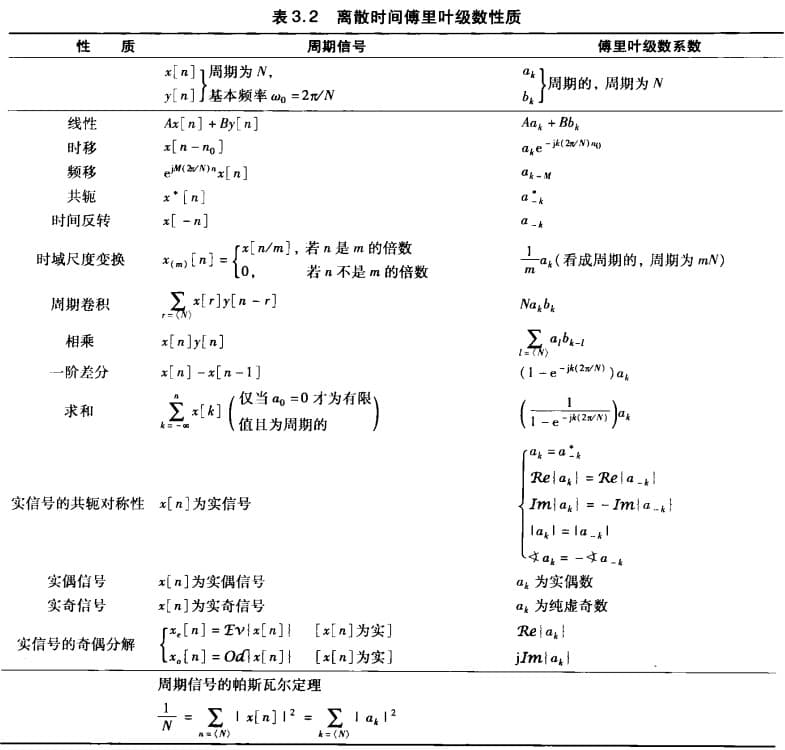# 离散时间傅里叶级数

\begin{align*} \newcommand{\dif}{\mathop{}\!\mathrm{d}} \newcommand{\xleftrightarrow}{\stackrel{#1}{\longleftrightarrow}} \end{align*}

# 离散时间周期信号的傅里叶级数

$k=\langle N \rangle$ 表示 $k$ 取 $1,2,\cdots N$。这是离散与连续的一大不同。因为 $k=N+1$ 对应的周期函数与 $k=1$ 是同一个。

# 离散傅里叶级数性质

## 相乘

$x[n],y[n]$ 是周期为 $N$ 的离散函数：

## 总结